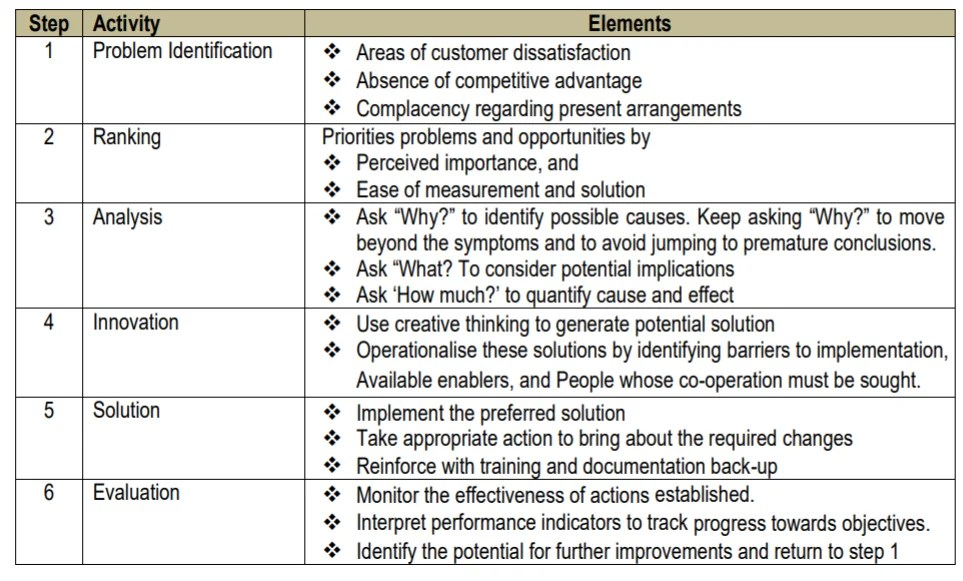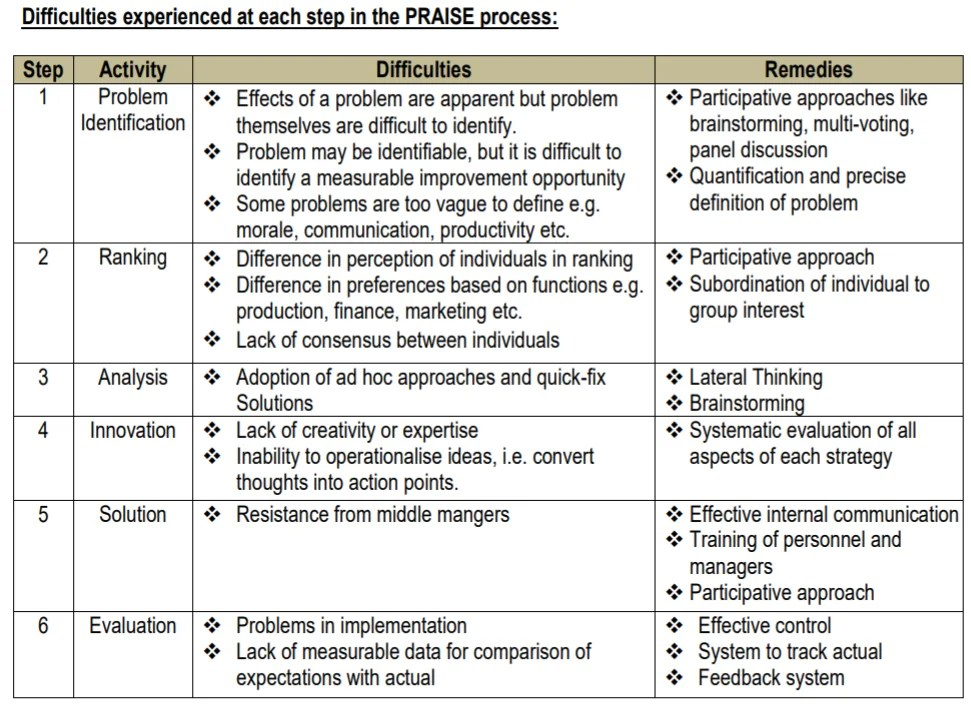# Praise Analysis and Simulation

28th February 2021

#### Praise Analysis

The identification of improvement opportunities and implementation of quality improvement process of the TQM Process is through a six-step activity sequence, identified by the acronym ‘PRAISE’. Following are the steps involved in PRAISE Analysis:#### Simulation

Simulation is a quantitative procedure which describes a process by developing a model of that process and then conducting a series of organized trial and error experiments to product the behaviour of the process over time. In practical life simulation technique is useful in following manner:

1. It is not possible to develop a mathematical model and solutions without some basic assumptions.
2. It may be too costly to actually observe a system.
3. Sufficient time may not be available to allow the system to operate for a very long time.
4. Actual operation and observation of a real system may be too disruptive.

Steps in the simulation process

1. Define the problem and system you intend to simulate.
2. Formulate the model you intend to use.
3. Test the model, compare with behaviour of the actual problem environment.
4. Identify and collect data to test the model.
5. Run the simulation.
6. Analyse the results of the simulation and, if desired, change the solution you are evaluating.
7. Rerun the simulation to tests the new solution.
8. Validate the simulation i.e., increase the chances of valid inferences.

1. The non-technical managers can comprehend simulation more easily than a complex mathematical model. Simulation does not require simplifications and assumptions to the extent required in analytical solutions. A simulation model is easier to explain to management personnel since it is a description of the behaviour of some system or process.
2. Simulation techniques allow experimentation with a model of the system rather than the actual operating system. Sometimes experimenting with the actual system itself could prove to be too costly and, in many cases too disruptive. For example, if you are comparing two ways of providing food service in a hospital, the confusion that would result from operating two different systems long enough to get valid observations might be too great. Similarly, the operation of a large computer central under a number of different operating alternatives might be too expensive to be feasible.
3. Simulation allows a user to analyze these large complex problems for which analytical results are not available. For example, in an inventory problem if the distribution for demand and lead time for an item follow a standard distribution, such as the poison distribution, then a mathematical or analytical solution can be found. However, when mathematically convenient distributions are not applicable to the problem, an analytical analysis of the problem may be impossible. A simulation model is a useful solution procedure for such problems.
4. Sometimes there is not sufficient time to allow the actual system to operate extensively. For example, if we were studying long-term trends in world population, we simply could not wait the required number of years to see results. Simulation allows the manger to incorporate time into an analysis. In a computer simulation of business operation, the manager can compress the result of several years or periods into a few minutes of running time.

Limitations:

1. Choice of random numbers is subjective.
2. Probability distribution based on past data may not reflect the future.
3. Number of iterations to achieve a desired result is a subjective affair.
4. It provides naïve approximations for significant decisions like capital budgeting, holding stock levels etc.
5. It is a time consuming and long exercise.
6. It generates a way of evaluating solutions but does not generate the solution itself.
7. All situations cannot be converted into simulation models.
8. Different iterations may provide different solutions and cause confusion to the evaluator.

The Monte Carlo Simulation:

1. Define the problem and select the measure of effectiveness of the problem that might be inventory shortages per period.
2. Identify the variables which influence the measure of effectiveness significantly for example, number of units in inventory.
3. Determine the proper cumulative probability distribution of each variable selected with the probability on vertical axis and the values of variables on horizontal axis.
4. Get a set of random numbers.
5. Consider each random number as a decimal value of the cumulative probability distribution with the decimal enter the cumulative distribution plot from the vertical axis. Project this point horizontally, until it intersects cumulative probability distribution curve. Then project the point of intersection down into the vertical axis.
6. Then record the value generated into the formula derived from the chosen measure o effectiveness. Solve and record the value. This value is the measure of effectiveness for that simulated value. Repeat above steps until sample is large enough for the satisfaction of the decision maker.